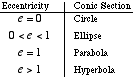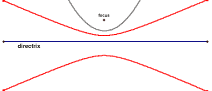eccentricity

Eccentricity of a Conic Section

A number that indicates how drawn out or attenuated a conic section is. Eccentricity is represented by the letter e (no relation to e = 2.718...). For horizontal ellipses and hyperbolas, eccentricity. For vertical ellipses and hyperbolas, eccentricity. Here, c = the distance from the center to a focus, a = the horizontal distance from the center to the vertex, and b = the vertical distance from the center to the vertex.Interactive FeatureConic Sections Defined by EccentricityJava Sketchpad version (Java enabled browser required)Geometer's Sketchpad version (GSP 4 required) Conic Sections Defined by Eccentricity 1. Move the slider for eccentricity and see what kind of conic section you get. 2. Move the focus to see what impact that has on the figure. 3. Move a point on the directrix to show that no matter what point is taken on the curve, the ratio of the distance to the focus over the distance to the directrix always equals the eccentricity.